# Basic functions - math word problems

1. CuboidsTwo separate cuboids with different orientation in space. Determine the angle between them, knowing the direction cosine matrix for each separate cuboid. u1=(0.62955056, 0.094432584, 0.77119944) u2=(0.14484653, 0.9208101, 0.36211633)
2. Points on line segmentPoints P & Q belong to segment AB. If AB=a, AP = 2PQ = 2QB, find the distance: between point A and the midpoint of the segment QB.
3. RemainderA is an arbitrary integer that gives remainder 1 in the division with 6. B is an arbitrary integer that gives remainder 2 the division by. What makes remainder in division by 3 product of numbers A x B ?
4. Trapezoid - intersection of diagonalsIn the ABCD trapezoid is AB = 8 cm long, trapezium height 6 cm, and distance of diagonals intersection from AB is 4 cm. Calculate trapezoid area.
5. Family 8Father is 38 years old, daughter 12, son 14. How many years will father have as many years as his children together?
6. Flowers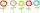The flower has six flowers and each flower has a number. These are the numbers: 20,40,39,28,8,9. What number will be in the middle of the flower so that the numbers come from the flowers when we subtract and add?
7. Photo enlarge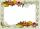Mark wants to enlarge a 4-inch by 6-inch photo so that it has a height of 15inches. Use a ratio table to determine the new width of the photo.
8. How far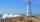From the top of a lighthouse 145 ft above sea level, the angle of depression of a boat 29°. How far is the boat from the lighthouse?
9. Clubhouse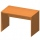There were only chairs and table in the clubhouse. Each chair had four legs, and the table was triple. Scouts came to the clubhouse. Everyone sat on their chair, two chairs were left unoccupied, and the number of legs in the room was 101. How many chairs w
10. Unknown numbersThe sum of two consecutive natural numbers and their triple is 92. Find these numbers.
11. Five pumpsThe water tank is filled with two pumps in 48 minutes. How long would it take to fill it with 5 same pumps?
12. CompetitorsIn the first round of slalom fell 15% of all competitors and in the second round another 10 racers. Together, 40% of all competitors fell. What was the total number of competitors?
13. Pie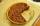Vili ate three pieces of pie . If it piece is 1/8 how much pie did he eat?
14. Unknown numberI think the number - its sixth is 3 smaller than its third.
15. ModuloFind x in modulo equation: 47x = 4 (mod 9) Hint - read as: what number 47x divided by 9 (modulo 9) give remainder 4 .
16. Boys and girlsThere are eight boys and nine girls in the class. There were six children on the trip from this class. What is the probability that left a) only boys b) just two boys
17. Photocopier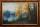A photocopier enlarges a picture in the ratio 7:4. How many times will a picture of size 6cm by 4cm be enlarged to fit on a 30cm by 20 cm page?
18. Fence and scaleThe garden with 80 m of fencing is draw on plan as a square with a side length of 4 cm. At what scale is this plan made?
19. Pie IIVili ate three pieces of pie. If it pieces is 1/8 how much pie did he eat?
20. SuzanSusan's age will be after 12 years four times as much as twelve years ago. How old is Susan now?

Do you have an interesting mathematical word problem that you can't solve it? Enter it, and we can try to solve it.

To this e-mail address, we will reply solution; solved examples are also published here. Please enter the e-mail correctly and check whether you don't have a full mailbox.

Please do not submit problems from current active competitions such as Mathematical Olympiad, correspondence seminars etc...